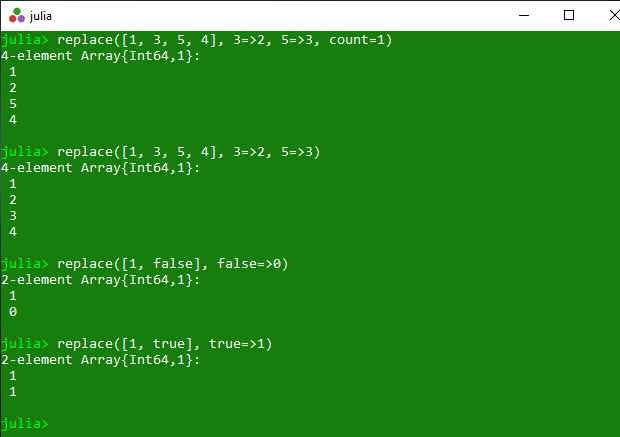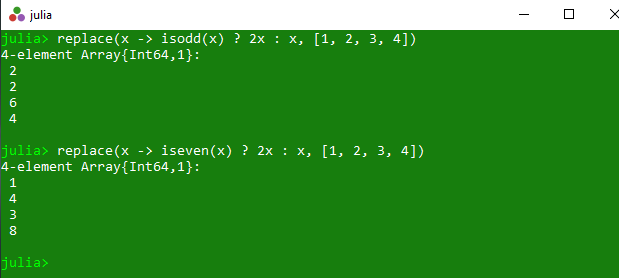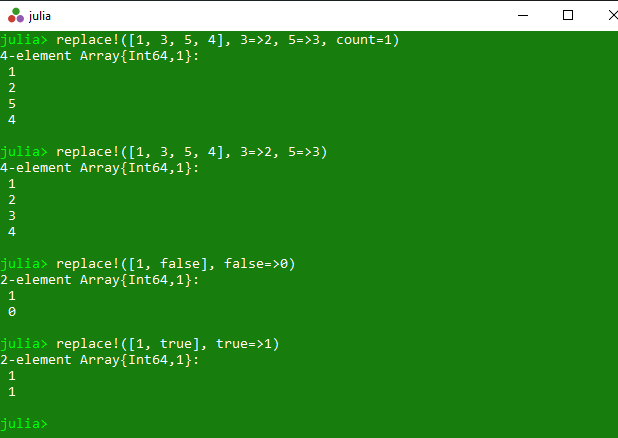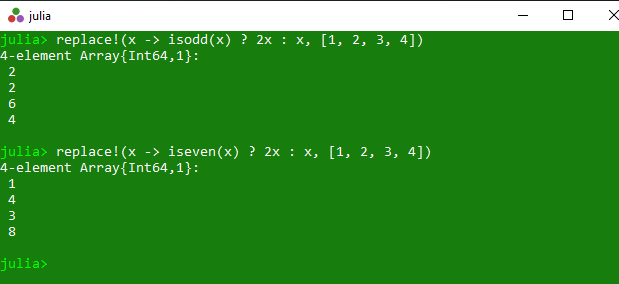# Replacing elements of a collection in Julia – replace() and replace!() Methods

The `replace()` is an inbuilt function in julia which is used to return a copy of the specified array with different types of replacement. These all operations are illustrated with below examples.

Syntax:
replace(A, old_new::Pair…; count::Integer)
or
replace(new::Function, A; count::Integer)

Parameters:

• A: Specified array.
• old_new::Pair: Specified values to get updated.
• count::Integer: This optional count value is used to replace at most count occurrences in total.
• new::Function: Specified set of instruction.

Returns: It returns a copy of the specified array with different types of replacement.

Example 1: This example returns a copy of the specified collection. In this collection old value get replaced with new value and if count is specified, then replace at most count occurrences in total.

 `# Julia program to illustrate  ` `# the use of replace() method ` `  `  `# Getting a copy of the specified collection.  ` `# In this collection old value get replaced  ` `# with new value and if count is specified,  ` `# then replace at most count occurrences in total. ` `println(replace([``1``, ``3``, ``5``, ``4``], ``3` `=``>``2``, ``5` `=``>``3``, count ``=` `1``)) ` `println(replace([``1``, ``3``, ``5``, ``4``], ``3` `=``>``2``, ``5` `=``>``3``)) ` `println(replace([``1``, false], false ``=``>``0``)) ` `println(replace([``1``, true], true ``=``>``1``)) `

Output:Example 2: This example returns a copy of a specified array where each value x in the array is replaced by new(x).

 `# Julia program to illustrate  ` `# the use of replace() method ` `  `  `# Getting a copy of a specified array  ` `# where each value x in the array is  ` `# replaced by new(x). ` `println(replace(x ``-``> isodd(x) ? ``2x` `: x, [``1``, ``2``, ``3``, ``4``])) ` `println(replace(x ``-``> iseven(x) ? ``2x` `: x, [``1``, ``2``, ``3``, ``4``])) `

Output:#### replace!()

The `replace!()` is an inbuilt function in julia which is used to return the replaced specified array with different types of replacement. These all operations are illustrated with below examples.

Syntax:
replace!(A, old_new::Pair…; count::Integer)
or
replace!(new::Function, A; count::Integer)

Parameters:

• A: Specified array.
• old_new::Pair: Specified values to get updated.
• count::Integer: This optional count value is used to replace at most count occurrences in total.
• new::Function: Specified set of instruction.

Returns: It returns the replaced array with different types of replacement method.

Example 1: This example returns the replaced collection. In this collection old value get replaced with new value and if count is specified, then replace at most count occurrences in total.

 `# Julia program to illustrate  ` `# the use of replace !() method ` `  `  `# Getting the replaced collection ` `# In this collection old value get replaced  ` `# with new value and if count is specified,  ` `# then replace at most count occurrences in total. ` `println(replace !([``1``, ``3``, ``5``, ``4``], ``3` `=``>``2``, ``5` `=``>``3``, count ``=` `1``)) ` `println(replace !([``1``, ``3``, ``5``, ``4``], ``3` `=``>``2``, ``5` `=``>``3``)) ` `println(replace !([``1``, false], false ``=``>``0``)) ` `println(replace !([``1``, true], true ``=``>``1``)) `

Output:Example 2: This example returns the replaced specified array where each value x in the array is replaced by new(x).

 `# Julia program to illustrate  ` `# the use of replace !() method ` `  `  `# Getting the replaced specified array  ` `# where each value x in the array is  ` `# replaced by new(x). ` `println(replace !(x ``-``> isodd(x) ? ``2x` `: x, [``1``, ``2``, ``3``, ``4``])) ` `println(replace !(x ``-``> iseven(x) ? ``2x` `: x, [``1``, ``2``, ``3``, ``4``])) `

Output:Whether you're preparing for your first job interview or aiming to upskill in this ever-evolving tech landscape, GeeksforGeeks Courses are your key to success. We provide top-quality content at affordable prices, all geared towards accelerating your growth in a time-bound manner. Join the millions we've already empowered, and we're here to do the same for you. Don't miss out - check it out now!